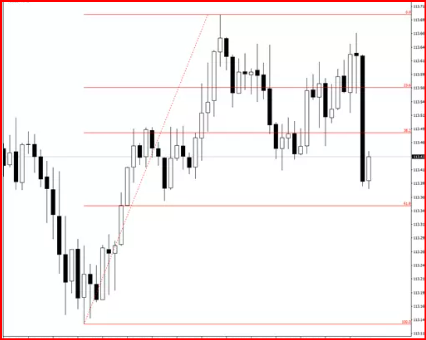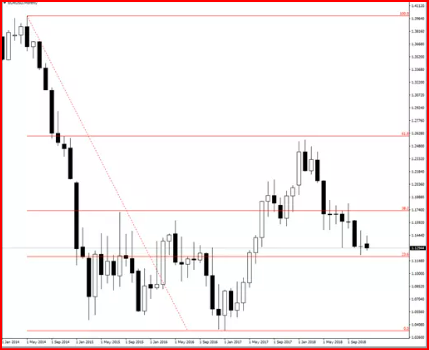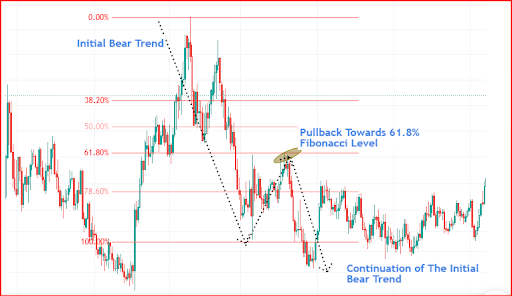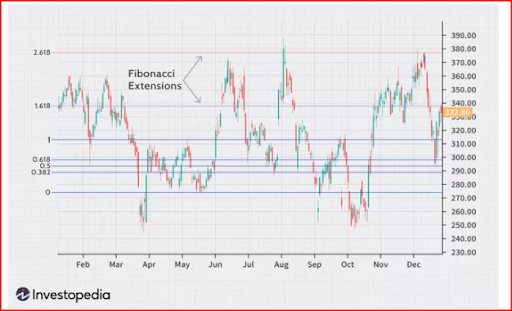## Fibonacci retracement and extensions

The Fibonacci Indicator is one of the popular indicators that is provided on most trading platforms. Leonard Pisano, who was a great mathematician of his time studied numbers and came up with the sequence known as the Fibonacci Sequence.

The sequence numbers developed by Leonardo Pisano are used in several trading styles and strategies. The sequence consists of 0,1 and then every number is the sum of two prior numbers: 0,1,1,2,3,5,8,13,21,34,55,89 and so on.

Using the numbers, Fibonacci Ratios are generated which helps in finding the Fibonacci retracements. In general, the Fibonacci retracement lines are formed using horizontal lines which indicates the reversal of a security’s price.

You need to understand the Fibonacci sequence numbers for understanding the retracement and extension prices. Further, you read this article that explains the Fibonacci trading strategies such as Fibonacci Extensions and Fibonacci Retracement and how fibonacci works.

## 1. Fibonacci Retracement

Fibonacci Retracement use in the stock market aims to indicate the reversal points with accuracy that helps traders to understand their trading aspects. The most common setting used in Fibonacci retracement is 23.6%, 38.2%, 61.8% and 78.6%.

The numbers used in the Fibonacci setting are the ratios that show the retracement levels. These levels are the utmost important development for traders while analysing the market.

Draw Fibonacci Retracement levels in an uptrend and downtrend as per the steps listed below:

1.1 Uptrend• First, identify the direction of the market which is an uptrend in this case.

• Select the Fibonacci Retracement tool that appears on the bottom, so drag it to right and then to the top.

• The three potential support levels 0.236,0.382, and 0.618 should be monitored.1.2 Downtrend• The direction of the market is identified as a downtrend.

• Attach the Fibonacci Retracement tool and drag it from the top to the right and the bottom.

• The three potential resistance levels 0.236, 0.382 and 0.618 should be monitored.

## 2. Application of Fibonacci Retracement

Fibonacci Levels are demanded by traders to trade perfectly with the support and resistance levels. A lot of traders dream to learn the Fibonacci Retracement theory and apply into their trading as soon as possible. Just learning the basics is not enough, as experience in trading using the tool is crucial for traders to start the application of the calculation.

The Fibonacci retracement levels come as handy when it is provided in trading platforms. A trader can use it to find the potential of the security price through uptrends and downtrends.

As a trading strategy, Fibonacci retracement is used in alignment with several trend trading strategies and trend continuations. Most traders use the golden ratio which is 61.8% to analyse the golden Fibonacci level. Here, the price is reversed and creates a new low which is attractive for the traders to enter.The above chart depicts the initial bear trend with the golden Fibonacci level which has a pullback and then a continuation of the bear trend. Traders identify these retracement levels while trading to become opportunists in such a situation.

Fibonacci Retracement levels are used to support a wide range of trading strategies. The aim is to find the potential where the reversal could happen in the chart. Most of the traders believe that the support and resistance developed in Fibonacci retracement levels are the trend continuation strategy.

For example, a trader notices a large impulse in the lower point and then notices a pullback price to the golden ratio (61.8%). In this situation, the trader decides to sell the security with the probability of bear movement being continued after the pullback. Thus, how Fibonacci retracement level helps traders to understand where the order should be entered in the possibility of a continuation trend.

## 3. Fibonacci Extensions

Fibonacci Extensions are a tool for traders to establish and estimate the profit targets or how far the price may go after a pullback. Extension levels are the areas which are used to predict where the price may reverse.

The Fibonacci Extensions are used to analyse the profit targets to know where to square off the trade. During an uptrend, the basic idea is to book the profits in the process of long trade with a Fibonacci Price Extension Level.

Fibonacci extensions do not have a specific formula, although when the indicator is applied, the trader selects three points. In the chart, the first point is the start of the movement, the second point is the end of a move, and the last point is the end of the retracement against the move.Extensions are drawn on a chart to mark the price levels for the entry and exit points. The levels are based on the Fibonacci ratios and the price moves as per these levels to help the traders indicate the price movements.

Fibonacci Extensions are extremely helpful for traders in determining backwards and forward prices to determine the strength and weaknesses of a few areas. Fibonacci extensions also help traders to find consistent support and resistance levels.

Some of the important points about Fibonacci Extensions a trader should know:

• Fibonacci Extension levels can be used in alignment with several strategies

• The most popular extension levels are 123.60%, 161.8%, 200% and 261.8%.

• These extensions are also used to validate some of the critically analysed points.

• Fibonacci Extension tools layout areas where the price may move ahead for the traders to identify.

## 4. Difference between Fibonacci Extensions and Fibonacci Retracements

Fibonacci retracement and extension are both used in the world of technical analysis concerning finding a price movement or area. Let’s get into the detailed differences that make them unique from each other

 Fibonacci Retracement Fibonacci Extension Fibonacci Retracement indicates how deep the reversal or retracement should take place. Fibonacci extension indicates the price movement and profit targets. It is used as a profitable strategy with other indicators. It measures the trend impulses and retrieves the direction of the trend. Fibonacci Retracement helps in providing good entry orders and stopping loss levels. Fibonacci Extension is used in developing a profit strategy with entry and exit points. The Fibonacci Numbers to analyse retracement are within the initial trend of 38.2%,61.8%, 50% etc. Extensions can help in pointing out good reversal points and good profit booking points. The Fibonacci Numbers to analyse the extension are beyond the 100% Fibonacci level i.e., 1.618%, 123.60% etc.## 5. Limitations of Fibonacci Retracement Levels

In technical analysis, as much as the indicators are useful, it possesses certain limitations as well. Trading in the stock markets comes with uncertainty with differences in market sentiment. Therefore, some of the limitations found in Fibonacci Retracement levels are listed below.

• Fibonacci Retracement level of 61.8% indicates that the security price will reverse from the point. However, it does not signify that price will reverse every time from that level as it depends upon more factors.

• Traders seem to stick to the Fibonacci retracement level strategies that work for them which can cause dilemmas among them. A retracement level that once worked is not necessarily would work the second time. It is crucial to understand the support, resistance, and retracement levels as per the security price to have an easy understanding of the Fibonacci numbers.

As the stock market is uncertain, the same goes for the indicators. Thus, a trader should be aware of the strategies used and discovered while trading to create an authentic setup.

Key Takeaways

• Fibonacci Sequence numbers should be identified before using them in the technical analysis.

• Fibonacci Retracement in the Uptrend direction appears on the bottom, and right and moves to the top.

• Fibonacci Retracement in a Downtrend direction appears on the top, and right and moves to the bottom.

• The golden ratio of 61.8% is used to analyse the potential retracements.

• Often, the pullback is generated after the golden ratio, however, the retracement cannot be formed every time. It depends upon certain other factors and analysis.

• Fibonacci Extensions help traders in determining profit targets, profit strategy, entry/exit points, and reversal points.

• Fibonacci Extension numbers are 1.618%, 123.60%

• Fibonacci Retracement numbers are 38.2%, 50%, 61.8%.

• Fibonacci extension points are three points, the first point is the start of the movement, the second point is the end of a move, and the last point is the end of the retracement against the move.